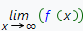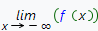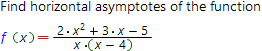# Horizontal asymptotes online calculatorHorizontal asymptote of the function f(x) called straight line parallel to x axis that is closely appoached by a plane curve. The distance between plane curve and this straight line decreases to zero as the f(x) tends to infinity. The horizontal asymptote equation has the form:

y = y0, where y0 - some constant (finity number)

To find horizontal asymptote of the function f(x), one need to find y0. To find the value of y0 one need to calculate the limitsandIf the value of both (or one) of the limits equal to finity number y0, then

y = y0 - horizontal asymptote of the function f(x).

To calculate horizontal asymptote of your function, you can use our free of charge online calculator, based on the Wolfram Aplha system.

Horizontal asymptotes calculatorFunction which horizontal asymptotes you want to find:

Install calculator on your site

## Other useful links:

Vertical asymptotes online calculator
Oblique asymptotes online calculator